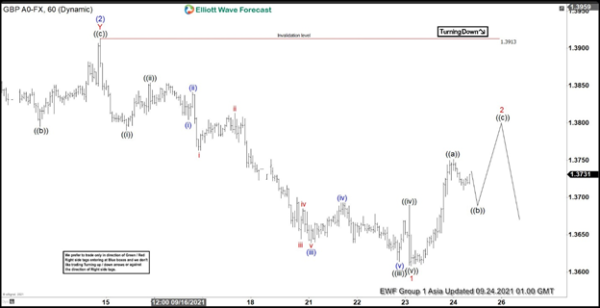# Elliott Wave View: GBP/USD Has Scope To Extend Correction

0
119A short-term view in GBPUSD suggests that the September 14, 2021 peak decline has developed as a structure of Elliott Wave’s 5-wave pulses. Below September 14, wave ((i)) ended at 1.3793 and accumulation in wave ((ii)) ended at 1.3854. A pair resumes lower in wave ((iii)) to 1.3616 with an internal subdivision as a 5-wave momentum to a lesser degree. Below wave ((ii)), wave (i) ended at 1.3806, and accumulation in wave (ii) ended at 1.3841. A pair resumes lower in wave (iii) to 1.3641 and accumulation in wave (iv) ended at 1.3693. Final leg lower wave (v) of ((iii)) ended at 1.3616.

Rally in wave ((iv)) ended at 1.3690 and turned lower in wave ((v)) ending at 1.3610 and completion wave 1. We are currently calling 3 waves to correct as wave 2. An inner subsection of wave 2 is developing as (( a)), ((b)), ((c)) zigzag structure. Wave ((a)) ended at 1.3751 and we are developing wave ((b)) in 3 swings which it has to bounce as wave ((c)) to complete wave 2. While we remain below 1.2913, we expected a further downside in the paro. . Near the term, until a pivot at 1.3913 highs remains intact, it is expected to fall in a 3, 7 or 11 swing for a further downside.

GBP / USD 60 Minutes Elliott Wave chartSource